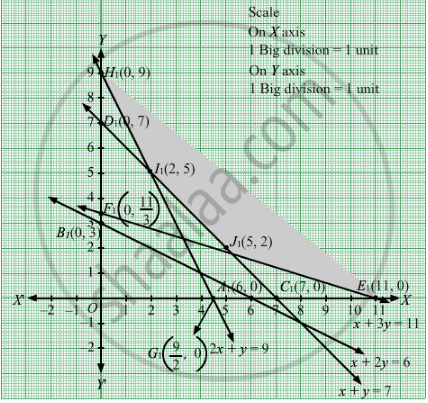# A Dietician Mixes Together Two Kinds of Food in Such a Way that the Mixture Contains at Least 6 Units of Vitamin A, 7 Units of Vitamin B, 11 Units of Vitamin C and 9 Units of Vitamin D. - Mathematics

Sum

A dietician mixes together two kinds of food in such a way that the mixture contains at least 6 units of vitamin A, 7 units of vitamin B, 11 units of vitamin and 9 units of vitamin D. The vitamin contents of 1 kg of food X and 1 kg of food Y are given below:

 VitaminA VitaminB VitaminC VitaminD Food XFood Y 12 11 13 21

One kg food X costs Rs 5, whereas one kg of food Y costs Rs 8. Find the least cost of the mixture which will produce the desired diet.

#### Solution

Let the dietician wishes to mix kg of food X and y kg of food Y.
Therefore,

$x, y \geq 0$

As we are given,

 Vitamin A Vitamin B Vitamin C Vitamin D Food XFood Y 12 11 13 21

It is given that the mixture should contain at least 6 units of vitamin A, 7 units of vitamin B, 11 units of vitamin C and 9 units of vitamin D.
Therefore, the constraints are

$x + 2y \geq 6$
$x + y \geq 7$
$x + 3y \geq 11$
$2x + y \geq 9$

It is given that cost of food X is Rs 5 per kg and cost of food Y is Rs 8 per kg.
Thus, Z =  $5x + 8y$

Thus, the mathematical formulat​ion of the given linear programmimg problem is
Minimize Z = $5x + 8y$

subject to

$x + 2y \geq 6$
$x + y \geq 7$
$x + 3y \geq 11$
$2x + y \geq 9$

First, we will convert the given inequations into equations, we obtain the following equations:
x + 2y = 6, x + y = 7x + 3y =11, 2x + y =9, x = 0 and y = 0.
The line x + 2y = 6 meets the coordinate axis at A1(6, 0) and B1(0, 3). Join these points to obtain the line x + 2y = 6. Clearly, (0, 0) does not satisfies the inequation x + 2y ≥ 6. So, the region in xy-plane that does not contains the origin represents the solution set of the given equation.
The line x + y = 7 meets the coordinate axis at C1(7, 0) and D1(0, 7). Join these points to obtain the line x + y = 7. Clearly, (0, 0) does not satisfies the inequation x + y ≥ 7. So, the region in xy-plane that does not contains the origin represents the solution set of the given equation.
The line x + 3y = 11 meets the coordinate axis at $E_1 \left( 11, 0 \right)$ and  $F_1 \left( 0, \frac{11}{3} \right)$ Join these points to obtain the line x + 3y = 11.Clearly, (0, 0) does not satisfies the inequation x + 3y ≥ 11. So, the region in xy-plane that does not contains the origin represents the solution set of the given equation.
The line 2x + y = 9 meets the coordinate axis at

$G_1 \left( \frac{9}{2}, 0 \right)$ and  $H_1 \left( 0, 9 \right)$ Join these points to obtain the line 2x + y = 9.Clearly, (0, 0) does not satisfies the inequation 2x + y ≥ 9. So, the region in xy-plane that does not contains the origin represents the solution set of the given equation.
Region represented by x ≥ 0 and y ≥ 0:
Since, every point in the first quadrant satisfies these inequations. So, the first quadrant is the region represented by the inequations.
These lines are drawn using a suitable scale.
The feasible region determined by the system of constraints isThe corner points are H1(0, 9), I1(2 ,5), J1(5, 2), E1(11, 0).
The values of Z at these corner points are as follows

 Corner point Z= 5x + 8y H1 72 I1 50 J1 41 E1 55

The minimum value of Z is at J1(5, 2) which is Rs 41.
Hence, cheapest combination of foods will be 5 units of food X and 2 units of food Y.

Concept: Graphical Method of Solving Linear Programming Problems
Is there an error in this question or solution?

#### APPEARS IN

RD Sharma Class 12 Maths
Chapter 30 Linear programming
Exercise 30.3 | Q 5 | Page 39